Share

# RD Sharma solutions for Class 8 Maths chapter 24 - Data Handling-II (Graphical Representation of Data as Histograms) [Latest edition]

Course
Textbook page

#### Chapters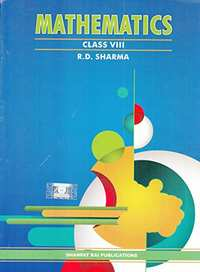## Chapter 24: Data Handling-II (Graphical Representation of Data as Histograms)

Ex. 24.1

#### RD Sharma solutions for Class 8 Maths Chapter 24 Data Handling-II (Graphical Representation of Data as Histograms) Exercise 24.1 [Pages 5 - 8]

Ex. 24.1 | Q 1 | Page 5

Given below is the frequency distribution of the heights of 50 students of a class:

 Class interval: 140−145 145−150 150−155 155−160 160−165 Frequency: 8 12 18 10 5

Draw a histogram representing the above data.

Ex. 24.1 | Q 2 | Page 5

Draw a histogram of the following data:

 Class interval: 10−15 15−20 20−25 25−30 30−35 34−40 Frequency: 30 98 80 58 29 50
Ex. 24.1 | Q 3 | Page 6

Number of workshops organized by a school in different areas during the last five years are as follows:

 Years No. of workshops 1995−1996 25 1996−1997 30 1997−1998 42 1998−1999 50 1999−2000 65

Draw a histogram representing the above data.

Ex. 24.1 | Q 4 | Page 6

In a hypothetical sample of 20 people the amounts of money with them were found to be as follows:
114, 108, 100, 98, 101, 109, 117, 119, 126, 131, 136, 143, 156, 169, 182, 195, 207, 219, 235, 118.
Draw the histogram of the frequency distribution (taking one of the class intervals as 50−100).

Ex. 24.1 | Q 5 | Page 6

Construct a histogram for the following data:

 Monthly school fee (in Rs): 30−60 60−90 90−120 120−150 150−180 180−210 210−240 Number of schools: 5 12 14 18 10 9 4
Ex. 24.1 | Q 6 | Page 6

Draw a histogram for the daily earnings of 30 drug stores in the following table:

 Daily earnings (in Rs): 450−500 500−550 550−600 600−650 650−700 Numbers of stores: 16 10 7 3 1
Ex. 24.1 | Q 7 | Page 6

Draw a histogram to represent the following data:

 Monthly salary (in Rs) Number of teachers 5600−5700 8 5700−5800 4 5800−5900 3 5900−6000 5 6000−6100 2 6100−6200 3 6200−6300 1 6300−6400 2
Ex. 24.1 | Q 8 | Page 7

The following histogram shows the number of literate females in the age group of 10 to 40 years in a town: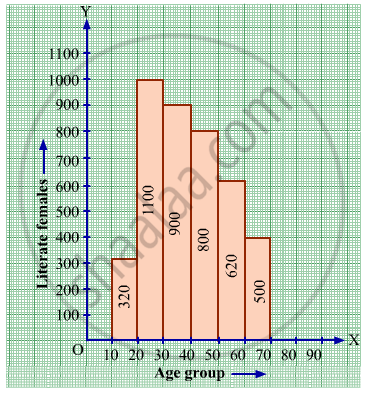(i) Write the age group in which the number of literate female is the highest.
(ii) What is the class width?
(iii) What is the lowest frequency?
(iv) What are the class marks of the classes?
(v) In which age group literate females are the least?

Ex. 24.1 | Q 9 | Page 7

The following histogram shows the monthly wages (in Rs) of workers in a factory: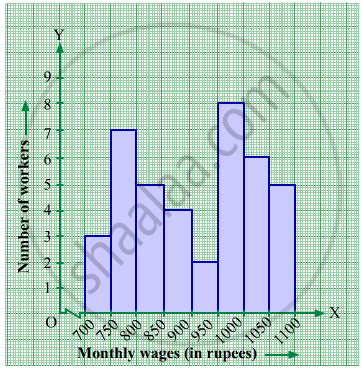(i) In which wage-group the largest number of workers are being kept? What is their number?
(ii)  What wages are the least number of workers getting? What is the number of such workers?
(iii) What is the total number of workers?
(iv) What is the factory size?

Ex. 24.1 | Q 10 | Page 8

Below is the histogram depicting marks obtained by 43 students of a class:
(i) Write the number of students getting the highest marks.
(ii) What is the class size?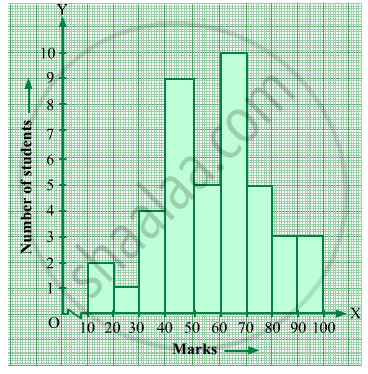Ex. 24.1 | Q 11 | Page 8

The following histogram shows the frequency distribution f the ages of 22 teachers in a school: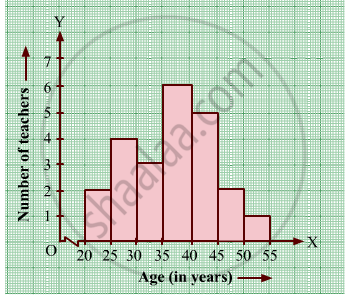(i) What is the number of eldest and youngest teachers in the school?
(ii) Which age group teachers are more in the school and which least?
(iii) What is the size of the classes?
(iv) What are the class marks of the classes?

Ex. 24.1 | Q 12 | Page 8

The weekly wages (in Rs.) of 30 workers in a factory are given:
830, 835, 890, 810, 835, 836, 869, 845, 898, 890, 820, 860, 832, 833, 855, 845, 804, 808, 812, 840, 885, 835, 835, 836, 878, 840, 868, 890, 806, 840
Mark a frequency table with intervals as 800-810, 810-820 and so on, using tally marks. Also, draw a histogram and answer the following questions:
(i) Which group has the maximum number of workers?
(ii) How many workers earn Rs 850 and more?
(iii) How many workers earn less than Rs 850?

## Chapter 24: Data Handling-II (Graphical Representation of Data as Histograms)

Ex. 24.1## RD Sharma solutions for Class 8 Maths chapter 24 - Data Handling-II (Graphical Representation of Data as Histograms)

RD Sharma solutions for Class 8 Maths chapter 24 (Data Handling-II (Graphical Representation of Data as Histograms)) include all questions with solution and detail explanation. This will clear students doubts about any question and improve application skills while preparing for board exams. The detailed, step-by-step solutions will help you understand the concepts better and clear your confusions, if any. Shaalaa.com has the CBSE Class 8 Maths solutions in a manner that help students grasp basic concepts better and faster.

Further, we at Shaalaa.com provide such solutions so that students can prepare for written exams. RD Sharma textbook solutions can be a core help for self-study and acts as a perfect self-help guidance for students.

Concepts covered in Class 8 Maths chapter 24 Data Handling-II (Graphical Representation of Data as Histograms) are Introduction of Grouping Data, Bars with a Difference, Introduction of Circle Graph Or Pie Chart, Circle Graph Or Pie Chart - Drawing Pie Charts, Chance and Probability - Getting a Result, Chance and Probability - Equally Likely Outcomes, Chance and Probability - Linking Chances to Probability, Chance and Probability - Outcomes as Events, Chance and Probability Related to Real Life, Organising Data, Looking for Information, Graphical Representation of Data as Histograms.

Using RD Sharma Class 8 solutions Data Handling-II (Graphical Representation of Data as Histograms) exercise by students are an easy way to prepare for the exams, as they involve solutions arranged chapter-wise also page wise. The questions involved in RD Sharma Solutions are important questions that can be asked in the final exam. Maximum students of CBSE Class 8 prefer RD Sharma Textbook Solutions to score more in exam.

Get the free view of chapter 24 Data Handling-II (Graphical Representation of Data as Histograms) Class 8 extra questions for Class 8 Maths and can use Shaalaa.com to keep it handy for your exam preparation